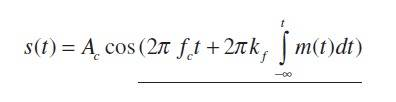# FM Signal Complex Envelope: Understanding & Resolving Integral

• FrankJ777
I understand it now. In summary, the FM complex envelope is given by: g(t) = Ae^{jθ(t)} where: A = |m(t)| and θ(t) = D_{f} \int^{t}_{-∞} m(σ) dσand if i want to determine the angle θ(t) of the FM complex envelope of the m(t) = Am cos (ωt) signal modulated by, i get: θ(t) = D_{f} \int^{t}

#### FrankJ777

Hey guys, I'm trying to understand the complex envelope of a Frequency Modulated signal. According to the Textbook by Leon Couch, the complex envelope of an FM signal, g(t) modulated by the signal m(t) is given by:

g(t) = Ae$^{jθ(t)}$

where

A = |m(t)|

and

θ(t) = D$_{f}$ $\int$$^{t}_{-∞}$ m(σ) dσ

Now if i want to determine the angle θ(t) of the complex envelope of the FM signal modulated by m(t) = Am cos (ωt)

i get:
θ(t) = D$_{f}$ $\int$$^{t}_{-∞}$ Am cos (ωσ) dσ

= D$_{f}$ Am $\frac{sin(ωσ)}{ω}$|$^{t}_{-∞}$

= D$_{f}$ Am ( $\frac{sin(ωt)}{ω}$ - $\frac{sin(ω(-∞)}{ω}$ ) This is where i get stuck. I'm not sure what to do with the -∞.

I though I knew how to deal with improper integrals, but I'm not sure where to go from here. Also I'm not sure why the lower limit of integration should be -∞, it seems to me like it should be zero? Any one know how I can resolve that integral? I would like to really understand this concept, but this part has me stuck.

Thanks a lot.

It should be 0 to t

Here is the equation for the FM complex envelope given by a tutorial from the Illinois Institute of Technology, also my textbook by Leon Couch uses an integral with the lower limit at negitive infinty. I did notice however that there are some tutorials that use 0 as the lower limit. Anyways, I'm not sure what to do with this.Here's a link to the whole tutorial.

http://www.ece.iit.edu/~biitcomm/research/references/Other/Tutorials%20in%20Communications%20Engineering/Tutorial%2017%20-%20Frequency%20Modulation%20(FM)%20,%20FSK,%20MSK%20and%20more.pdf

I'll take a stab at it, but I'm not an expert. Since, in the above equation, the intregal represents the phase, you must integrate the frequency since the beginning of time to determine the phase. Since you are dealing with a sinewave and it repeats you can assume an arbitrary starting time. I'm not sure about the difference between starting at 0 or pi/4 or any other initial time. But I think you just exploit the periodic nature of the signal.

I read the whole tutorial: it tries to keep things simple, which is a good (since it is just an introductory tutorial), but I think this may lead to some inconsistencies if you aren't careful. However, I noted a slightly abused notation; thus, let me restate things a bit.

Given the following signal: $s(t) = A_c\cos(2\pi f_c t + \varphi(t))$, you can define the so-called instantaneous phase $\theta(t) \triangleq 2\pi f_c t + \varphi(t)$, and the so-called instantaneous frequency $f_i(t) = \frac{1}{2\pi} \frac{d}{dt} \theta(t)$. We know that in FM: $k_fm(t)=\frac{1}{2\pi} \frac{d}{dt} \varphi(t)$. Now, if you try to solve this equation, giving an explicit expression of $\varphi(t)$, you end up with the integral you wrote. Usually, that integral goes from $t_0$ (which can be set equal to zero) to a generic $t$. However, it isn't technically incorrect to say that it goes from $-\infty$ to $t$ if you let $m(t)$ be a causal signal, i.e. there must exist $t_0$ (which, again, can be zero) such as that $m(t)=0 \ \ for \ \ t<t_0$. More precisely, the generic expression is: $$\varphi(t) = 2\pi k_f \int^t_{t_0} m(\tau) d\tau + \varphi_0$$ where $\varphi_0 \triangleq \varphi(t_0)$ is the initial phase (which is irrelevant and can be neglected). By choosing $t_0=0$ and $\varphi_0=0$, you obtain:
$$\varphi(t) = 2\pi k_f \int^t_0 m(\tau) d\tau$$ and this is the standard formula. If you (re)do all the computations with this formula, you can "forget" the fact that $m(t)$ must be causal, since the integration domain is now a finite time interval. However, if you want to keep using your notation, then you must write: $m(t)=A_m cos(\omega t) u(t)$, where u(t) is the unit step function (zero for t<0, 1 otherwise), where I assumed (for simplicity) $t_0=0$. Hope this helps.

Nice explanation.

Thanks for your guy's explanations. If i can sum up what you said...
When we consider signals, m(t) that begin at time t=0 or t> 0, which are realizable signals, we evaluate the integral from the lower limit of 0?
That seems to make sense to me. Hope I understand it correctly.
Thanks.

So the integral with the lower limit of neg infinity, is a more general expression, that could include non causal signals?

Last edited:
FrankJ777 said:
So the integral with the lower limit of neg infinity, is a more general expression, that could include non casual signals?
You mean "causal", right? Yes, it may be thought as such (theoretically).

meBigGuy said:
Nice explanation.
Thanks!

Oops! Causul. Just edited it!
Thanks.